## CONFORMAL

 Applies a conformal mapping to an image

 USAGE: conformal [-d dimensions] [-f formula] [-g gain] [-v vp] [-b bcolor] [-z zcolor] [-m monitor] infile outfile USAGE: conformal [-h|-help] -d ... dimension ... output dimensions WxH; "x" separated pair of integers>0; .................... default=input dimension -f ... formula ..... flag for the complex expression; integer between .................... 1 and 8; default=1 -g ... gain ........ effect gain factor (zoom); pair of comma separate .................... floats>0; xgain,ygain; default="1,1" -v ... vp .......... virtual-pixel method; default=tile -b ... bcolor ...... background color for virtual-pixel method; any valid .................... IM color is allowed; default=black -z ... zcolor ...... zero color; color for where the formula has a divide .................... by zero; default=black -m ... monitor ..... monitor enables the progress monitor; choices are: .................... on or off; default=off PURPOSE: To apply a conformal mapping to an image. DESCRIPTION: CONFORMAL applies a conformal mapping to an image. The user may select from on of 7 complex expressions. The virtual pixel method may also be selected. ARGUMENTS: -d dimension ... DIMENSIONS specify the output image dimensions WxH. The value is an "x" separated pair of integers>0. The default=input dimensions -f formula ... FORMULA is a flag for the complex expression. Value are integer between 1 and 8. 1=-1/z, 2=(z+1/z)/2, 3=z^2, 4=sin(z), 5=cos(z), 6=ln(z), 7=(z+1)/(z-1), 8=1/(4*z^2-1), where z=x+i*y is a complex value. The default=1. -g gain ... GAIN is a gain factor (zoom) that controls the look of the conformal mapping. Values are a pair of comma separate floats>0 as "xgain,ygain". If only one value is provided it will be used for both. The default="1,1". -v vp ... VP is the virtual-pixel method. Any virtual-pixel method is allowed. The default=tile. -b bcolor ... BCOLOR is the background color for the virtual-pixel method that is a color. Any valid IM color is allowed. The default=black. -z zcolor ... ZCOLOR is the zero color, which is the color for where the formula has a divide by zero. Relevant only to formula 1, 2 and 6. The default=black. -m monitor ... MONITOR enables the progress monitor. Choices are: on or off. The default=off NOTE: This script will be slow due to the use of -fx. On my Mac Mini with two cores, it took about 10-15 sec for a 500x500 image. CAVEAT: No guarantee that this script will work on all platforms, nor that trapping of inconsistent parameters is complete and foolproof. Use At Your Own Risk.

### EXAMPLES

 Example 1 -- Formula 1 -- -1/z Original Image (source)Arguments: -f 1 -g 1 -v tileArguments: -f 1 -g 1 -v blackArguments: -f 1 -g 0.5 -v tileArguments: -f 1 -g 2 -v tileArguments: -f 1 -g 3 -v tileExample 2 -- Formula 2 -- (z+1/z)/2 Original Image (source)Arguments: -f 2 -g 1 -v tile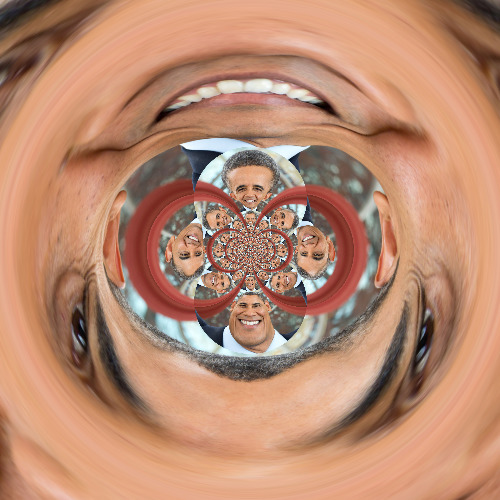Arguments: -f 2 -g 1 -v blackArguments: -f 2 -g 0.5 -v tileArguments: -f 2 -g 2 -v tileArguments: -f 2 -g 3 -v tileExample 3 -- Formula 3 -- z^2 Original Image (source)Arguments: -f 3 -g 1 -v tileArguments: -f 3 -g 1 -v black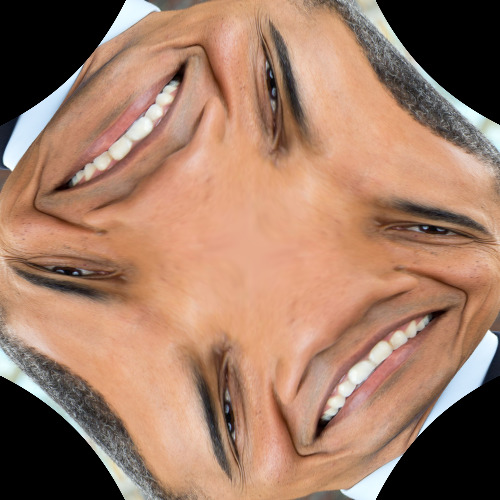Arguments: -f 3 -g 2 -v tileArguments: -f 3 -g 3 -v tileExample 4 -- Formula 4 -- sin(z) Original Image (source)Arguments: -f 4 -g 1 -v tileArguments: -f 4 -g 0.5 -v tileArguments: -f 4 -g 2 -v tileArguments: -f 4 -g 2 -v black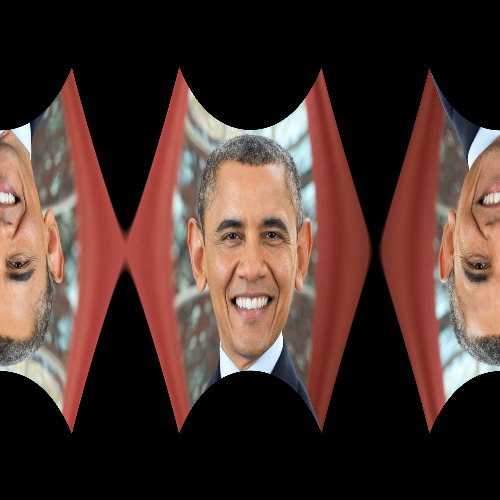Example 5 -- Formula 5 -- cos(z) Original Image (source)Arguments: -f 5 -g 1 -v tileArguments: -f 5 -g 0.5 -v tile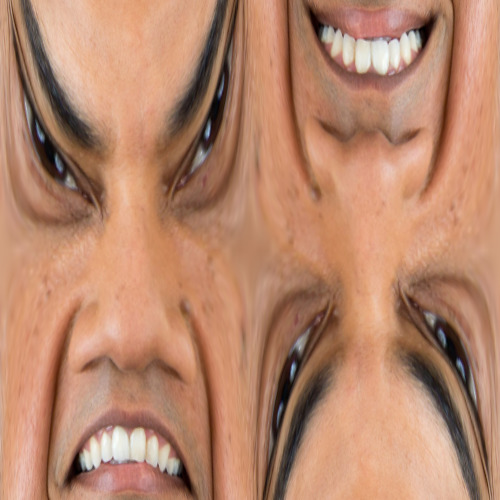Arguments: -f 5 -g 2 -v tileArguments: -f 5 -g 2 -v blackExample 6 -- Formula 6 -- ln(z) Original Image (source)Arguments: -f 6 -g 1 -v tileArguments: -f 6 -g 1 -v blackArguments: -f 6 -g 0.5 -v tileArguments: -f 6 -g 2 -v tileArguments: -f 6 -g 3 -v tileExample 7 -- Formula 7 -- (z+1)/(z-1) Original Image (source)Arguments: -f 7 -g 1 -v tileArguments: -f 7 -g 1 -v blackArguments: -f 7 -g 0.5 -v tileArguments: -f 7 -g 2 -v tileArguments: -f 7 -g 3 -v tile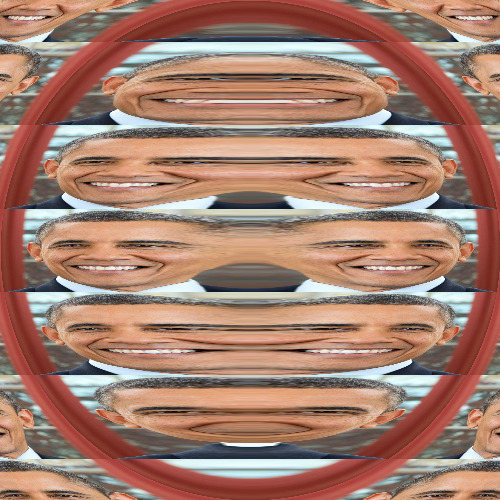Example 8 -- Formula 8 -- 1/(4*z^2-1) Original Image (source)Arguments: -f 8 -g 1 -v tile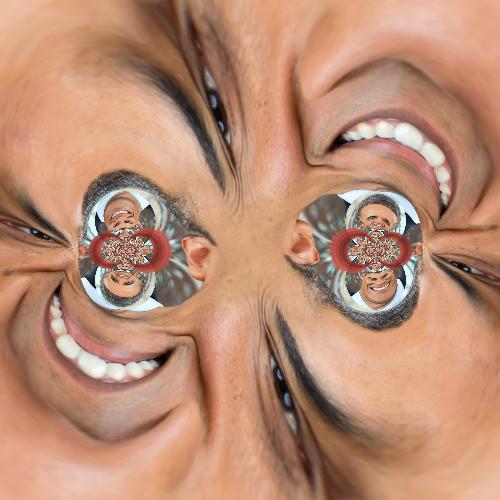Arguments: -f 8 -g 1 -v black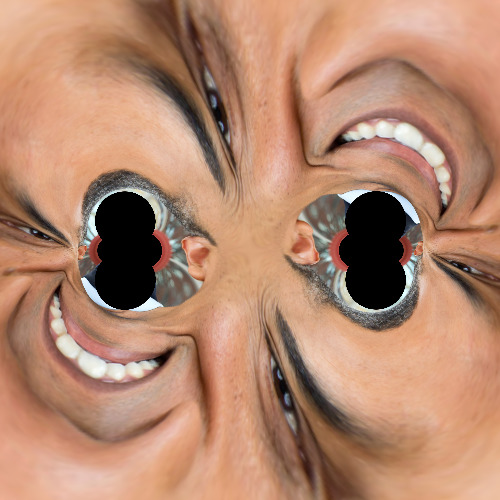Arguments: -f 8 -g 0.5 -v tileArguments: -f 8 -g 2 -v tileArguments: -f 8 -g 3 -v tileWhat the script does is as follows: Reads the input image and computes size related arguments Selects the conformal map formula and computes the appropriate -fx expression Writes the output This is equivalent to the following IM commands: wxh=`convert -ping \$dir/tmpI.mpc -format "%wx%h" info:` ww=`echo "\$wxh" | cut -dx -f1` hh=`echo "\$wxh" | cut -dx -f2` ww2=`convert xc: -format "%[fx:\$ww/2]" info:` hh2=`convert xc: -format "%[fx:\$hh/2]" info:` ww4=`convert xc: -format "%[fx:\$ww/4]" info:` hh4=`convert xc: -format "%[fx:\$hh/4]" info:` if [ "\$dimensions" = "" ]; then wd=\$ww ht=\$hh else wd=`echo "\$dimensions" | cut -dx -f1` ht=`echo "\$dimensions" | cut -dx -f2` fi wd2=`convert xc: -format "%[fx:\$wd/2]" info:` ht2=`convert xc: -format "%[fx:\$ht/2]" info:` wd4=`convert xc: -format "%[fx:\$wd/4]" info:` ht4=`convert xc: -format "%[fx:\$ht/4]" info:` gx=`echo "\$gain" | cut -d, -f1` gy=`echo "\$gain" | cut -d, -f2` if [ "\$monitor" = "on" ]; then monitoring1="-monitor" monitoring2="+monitor" else monitoring1="" monitoring2="" fi if [ \$formula -eq 1 ]; then convert -size \${wd}x\${ht} xc: \$dir/tmpI.mpc \ -background "\$bcolor" -virtual-pixel \$vp \$monitoring1 -fx \ "xx=(i-\$wd2)/\$wd2; yy=(j-\$ht2)/\$ht2; rr=(xx*xx+yy*yy); \ (xx==0 && yy==0)?\$zcolor:v.p{-\$gx*\$ww4*xx/rr+\$ww2,\$gy*\$hh4*yy/rr+\$hh2}" \v \$monitoring2 "\$outfile" elif [ \$formula -eq 2 ]; then convert -size \${wd}x\${ht} xc: \$dir/tmpI.mpc \ -background "\$bcolor" -virtual-pixel \$vp \$monitoring1 -fx \ "xx=(i-\$wd2)/\$wd2; yy=(j-\$ht2)/\$ht2; rr=(xx*xx+yy*yy); \ (xx==0 && yy==0)?\$zcolor:v.p{\$gx*\$ww4*(xx*rr-xx)/rr+\$ww2,\$gy*\$ww4(yy*rr-yy)/rr+\$hh2}" \ \$monitoring2 "\$outfile" elif [ \$formula -eq 3 ]; then convert -size \${wd}x\${ht} xc: \$dir/tmpI.mpc \ -background "\$bcolor" -virtual-pixel \$vp \$monitoring1 -fx \ "xx=(i-\$wd2)/\$wd2; yy=(j-\$ht2)/\$ht2; \ v.p{\$gx*\$ww4*(xx*xx-yy*yy)+\$ww2,\$gy*\$hh2*2*xx*yy+\$hh2}" \ \$monitoring2 "\$outfile" elif [ \$formula -eq 4 ]; then convert -size \${wd}x\${ht} xc: \$dir/tmpI.mpc \ -background "\$bcolor" -virtual-pixel \$vp \$monitoring1 -fx \ "xx=(i-\$wd2)/\$wd2; yy=(j-\$ht2)/\$ht2; \ v.p{\$gx*\$ww4*sin(pi*xx)*cosh(yy)+\$ww2,\$gy*\$hh2*cos(pi*xx)*sinh(yy)+\$hh2}" \ \$monitoring2 "\$outfile" elif [ \$formula -eq 5 ]; then convert -size \${wd}x\${ht} xc: \$dir/tmpI.mpc \ -background "\$bcolor" -virtual-pixel \$vp \$monitoring1 -fx \ "xx=(i-\$wd2)/\$wd2; yy=(j-\$ht2)/\$ht2; \ v.p{\$gx*\$ww4*cos(pi*xx)*cosh(yy)+\$ww2,-\$gy*\$hh2*sin(pi*xx)*sinh(yy)+\$hh2}" \ \$monitoring2 "\$outfile" elif [ \$formula -eq 6 ]; then convert -size \${wd}x\${ht} xc: \$dir/tmpI.mpc \ -background "\$bcolor" -virtual-pixel \$vp \$monitoring1 -fx \ "xx=(i-\$wd2)/\$wd2; yy=(j-\$ht2)/\$ht2; rr=(xx*xx+yy*yy); \ ( (rr==0) || (xx==0) )?\$zcolor:v.p{\$gx*\$ww4*ln(sqrt(rr))+\$ww2,\$gy*\$hh2*atan(yy/xx)+\$hh2}" \ \$monitoring2 "\$outfile" fi